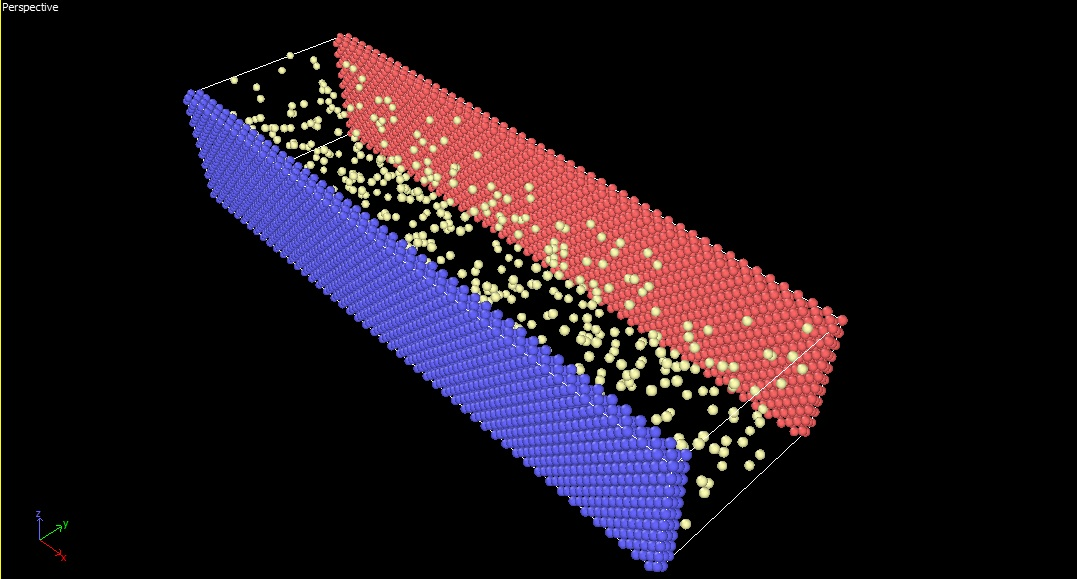# volume for “compute heat/flux command”

Hi everyone

Actually I am using “heat/flux command” in LAMMPS to calculate the heat flux between two stationary parallel walls that the region between them is filled with a gas. I implement “heat/flux command” on gas region to obtain the heat flux which pass through that.

According to LAMMPS manual, I use the following line to calculate the heat flux through the gas (flow group is assigned to the volume which is located between two walls and filled with gas atoms which can move freely between walls)Hi everyone

Actually I am using “heat/flux command” in LAMMPS to calculate the heat flux
between two stationary parallel walls that the region between them is filled
with a gas. I implement “heat/flux command” on gas region to obtain the heat
flux which pass through that.

According to LAMMPS manual, I use the following line to calculate the heat
flux through the gas (flow group is assigned to the volume which is located
between two walls and filled with gas atoms which can move freely between
walls)

###########################################
compute myKE flow ke/atom
compute myPE flow pe/atom
compute myStress flow stress/atom NULL virial
compute flux flow heat/flux myKE myPE myStress
variable Jx equal c_flux/\$V
variable Jy equal c_flux/\$V
variable Jz equal c_flux/\$V
###########################################

In LAMMPS manual (compute heat/flux command part), heat flux through the
solid argon was calculated in similar way. In this method, Jx = flux /\$V
and the in manual, \$V is considered as the volume of simulation box because
in that case, all the simulation box was filled with argon.

But in my case, the gas doesn’t fill the gap between two walls completely
(actually I want to model the rarefied gas)
So I just want to know the exact value of the \$V in my case. Should I
consider the whole volume of flow group?

what is a "rarefied gas"?
noble gas? low density gas?

Or as the gas is rarefied which doesn’t fill the whole space between the
walls completely, I should only consider a portion of the volume between two
walls? (if this is the case, how can I calculate the exact value for \$V?)

In “compute heat/flux command” parts of the manual, it was written that if a
sub-group of simulation box is used in compute command, \$V is the volume of
that group only (not the whole simulation box). But I can’t find any hint
about the case like mine that in such sub-group, the gas atoms don’t fill
the whole region completely.

volume is volume, independent of the density of the material.

before studying an as complex system as you do, i would recommend that
you first gain experience by studying a simpler, bulk system (with
normal density). then i would suggest you study the literature, to
find out if the methodology used in compute heat/flux is actually
applicable to non-condensed systems, what kind of error bars to
expect, what amount of statistical sampling is required and then try
to reproduce some data for simple bulk 1 component systems before
moving on to study your complex setup.

for that kind of system, you have to be aware that volume of
subsystems, is not a very well defined quantity on the atomic scale.
there are numerous discussions on the subject in the mailing list
archive and multiple possible strategies for different kinds of setups
that provide a more or less reliable approximation.

at any rate, these are really more conceptual questions and not very
specific to LAMMPS.

axel.

heat/flux command is based on the heat theorem. It is a volume averaged quantity in the sense that the value represents the average of the heat flux vector in the encompassed volume of atomic interactions you care about. Are you just interested in measuring the average heat flux that is experienced by the gas in between the two walls? or the average heat flux from one wall to the next through the gas? If you want the latter the volume must include the walls as well. On another note, the value won’t be a good representative of the heat flux unless you’re system is in steady state.

Thank you so much for your consideration.
Just one other thing. Do you know where can I found additional information about heat theorem? Do you know whether this theory is applicable for gas in different density or not?

Reza

Dear Alex,

Thank you so much for your consideration and suggestion.

Actually I am using LAMMPS to model rarefied gas in micro and nano channels.

Just one other question. Is there any other method available in LAMMPS to compute heat flux except “compute heat/flux command”? Actually I found some NEMD method for calculating thermal conductivity but about the heat flux, I didn’t find any other thing.

Thank you!
Reza